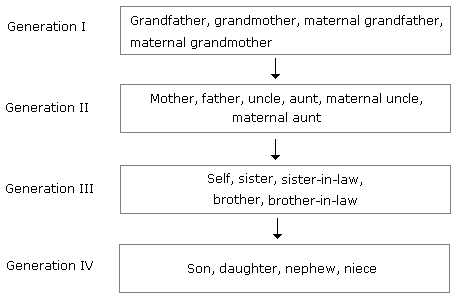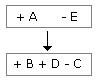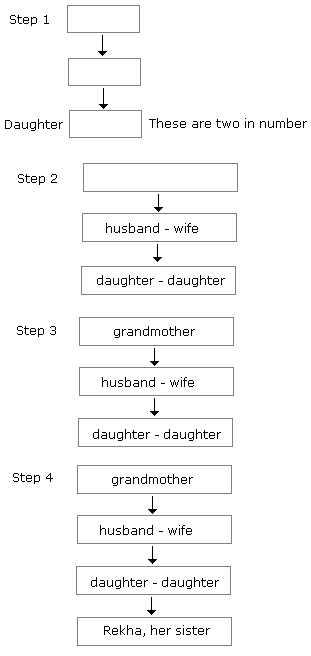# Verbal Reasoning - Blood Relation Test

### Exercise :: Blood Relation Test - Introduction

Introduction:

The questions which are asked in this section depend upon Relation. You should have a sound knowledge of the blood relation in order to solve the questions.

To remember easily the relations may be divided into two sides as given below:

1. Relations of Paternal side:

1. Father's father → Grandfather
2. Father's mother → Grandmother
3. Father's brother → Uncle
4. Father's sister → Aunt
5. Children of uncle → Cousin
6. Wife of uncle → Aunt
7. Children of aunt → Cousin
8. Husband of aunt → Uncle

2. Relations of Maternal side:

1. Mother's father → Maternal grandfather
2. Mother's mother → Maternal grandmother
3. Mother's brother Maternal uncle
4. Mother's sister → Aunt
5. Children of maternal uncle → Cousin
6. Wife of maternal uncle → Maternal aunt

Relations from one generation to next:Differenct types of questions with explanation:

Type 1:

If A + B means A is the mother of B; A x B means A is the father of B; A \$ B means A is the brother of B and A @ B means A is the sister of B then which of the following means P is the son of Q?

(A) Q + R @ P @ N       (B) Q + R * P @ N

(C) Q x R \$ P @ N        (D) Q x R \$ P \$ N

Solution: (D)

Q x R = Q is the father of R [-Q, ±R]

R \$ P = R is the brother of P [+ R, ±P]

P \$ N = P is the brother of N [+ P, ±N]

Therefore P is the son of Q.

Type 2:

A has 3 children. B is the brother of C and C is the sister of D, E who is the wife of A is the mother of D. There is only one daughter of the husband of E. what is the relation between D and B?

Solution: With the chartTherefore, D is a boy because there is only one daughter of E.

Hence, B is the brother of D.

Type 3:

Pointing to a photograph, Rekha says to Lalli, "The girl in the photo is the second daughter of the wife of only son of the grandmother of my younger sister." How this girl of photograph is related to Rekha?

Solution:

First Method - By Generating Charts:Second method:

1. Grandmother of younger sister of Rekha → Grandmother of Rekha
2. Wife of only son of grandmother → Mother of Rekha
3. Younger daughter of the mother → Younger sister.

Note: While solving the question (+) can be used for male and (-) can be used for female.

#### Current Affairs 2022

Interview Questions and Answers